# waveguideCircular

Create circular waveguide

Since R2019b

## Description

The `waveguideCircular` object creates a circular waveguide. A circular waveguide is a hollow tube of uniform cross section, that confines the electromagnetic wave. This antenna is used in radar and short and medium distance broadband communication.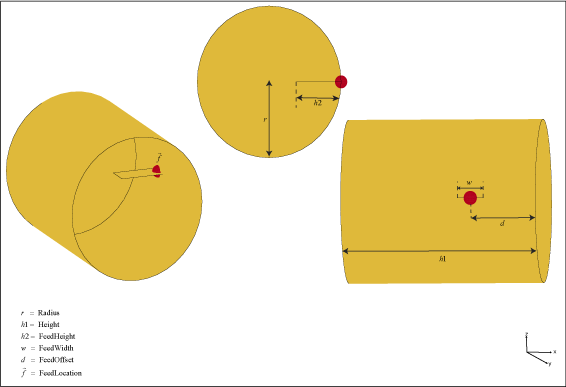## Creation

### Syntax

``ant = waveguideCircular``
``ant = waveguideCircular(Name,Value)``

### Description

example

````ant = waveguideCircular` creates a circular waveguide with dimensions for an operating frequency of 8.42 GHz.```

example

````ant = waveguideCircular(Name,Value)` sets properties using one or more name-value pairs. For example, `ant = waveguideCircular('Height',1)` creates a circular waveguide with a height of 1 meter.```

## Properties

expand all

Height of the circular waveguide, specified as a real-valued scalar in meters.

Example: `'Height',0.0215`

Example: `ant.Height = 0.0215`

Data Types: `double`

Radius of the circular waveguide, specified as a real-valued scalar in meters.

Example: `'Radius',0.0546`

Example: `ant.Radius = 0.0546`

Data Types: `double`

Height of the feed, which is equal to the height of the monopole, specified as a real-valued scalar in meters.

Example: `'FeedHeight',0.0034`

Example: `ant.FeedHeight = 0.0034`

Data Types: `double`

Width of the feed, which is equal to the width of the monopole, specified as a real-valued scalar in meters.

Example: `'FeedWidth',0.0050`

Example: `ant.FeedWidth = 0.0050`

Data Types: `double`

Vertical distance of the feed along the Y-axis, specified as a real-valued scalar in meters.

Example: `'FeedOffset',0.0050`

Example: `ant.FeedOffset = 0.0050`

Data Types: `double`

Type of the metal used as a conductor, specified as a metal material object. You can choose any metal from the `MetalCatalog` or specify a metal of your choice. For more information, see `metal`. For more information on metal conductor meshing, see Meshing.

Example: ```m = metal('Copper'); 'Conductor',m```

Example: ```m = metal('Copper'); ant.Conductor = m```

Lumped elements added to the antenna feed, specified as a lumped element object handle. You can add a load anywhere on the surface of the antenna. By default, the load is at the feed. For more information, see `lumpedElement`.

Example: `'Load',lumpedelement`. `lumpedelement` is the object handle for the load created using `lumpedElement`.

Example: ```ant.Load = lumpedElement('Impedance',75)```

Tilt angle of the antenna in degrees, specified as a scalar or vector. For more information, see Rotate Antennas and Arrays.

Example: `90`

Example: `Tilt=[90 90]`,`TiltAxis=[0 1 0;0 1 1]` tilts the antenna at 90 degrees about the two axes defined by the vectors.

Data Types: `double`

Tilt axis of the antenna, specified as one of these values:

• Three-element vector of Cartesian coordinates in meters. In this case, each coordinate in the vector starts at the origin and lies along the specified points on the x-, y-, and z-axes.

• Two points in space, specified as a 2-by-3 matrix corresponding to two three-element vectors of Cartesian coordinates. In this case, the antenna rotates around the line joining the two points.

• `"x"`, `"y"`, or `"z"` to describe a rotation about the x-, y-, or z-axis, respectively.

Example: `[0 1 0]`

Example: `[0 0 0;0 1 0]`

Example: `"Z"`

Data Types: `double` | `string`

## Object Functions

 `show` Display antenna, array structures, shapes, or platform `axialRatio` Axial ratio of antenna `beamwidth` Beamwidth of antenna `charge` Charge distribution on antenna or array surface `current` Current distribution on antenna or array surface `design` Design prototype antenna or arrays for resonance around specified frequency or create AI-based antenna from antenna catalog objects `efficiency` Radiation efficiency of antenna `EHfields` Electric and magnetic fields of antennas or embedded electric and magnetic fields of antenna element in arrays `impedance` Input impedance of antenna or scan impedance of array `mesh` Mesh properties of metal, dielectric antenna, or array structure `meshconfig` Change meshing mode of antenna, array, custom antenna, custom array, or custom geometry `optimize` Optimize antenna or array using SADEA optimizer `pattern` Plot radiation pattern and phase of antenna or array or embedded pattern of antenna element in array `patternAzimuth` Azimuth plane radiation pattern of antenna or array `patternElevation` Elevation plane radiation pattern of antenna or array `rcs` Calculate and plot radar cross section (RCS) of platform, antenna, or array `returnLoss` Return loss of antenna or scan return loss of array `sparameters` Calculate S-parameters for antennas and antenna arrays `vswr` Voltage standing wave ratio (VSWR) of antenna or array element

## Examples

collapse all

Create and view a default circular waveguide.

`ant = waveguideCircular`
```ant = waveguideCircular with properties: Radius: 0.0120 Height: 0.0300 FeedHeight: 0.0075 FeedWidth: 0.0040 FeedOffset: 0.0100 Conductor: [1x1 metal] Tilt: 0 TiltAxis: [1 0 0] Load: [1x1 lumpedElement] ```
`show(ant)`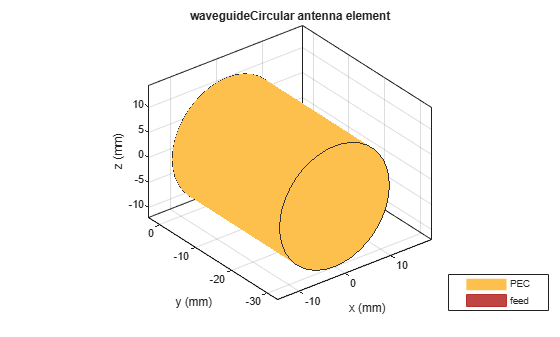Plot the radiation pattern of the antenna at 7.42 GHz.

`pattern(ant,7.42e9)`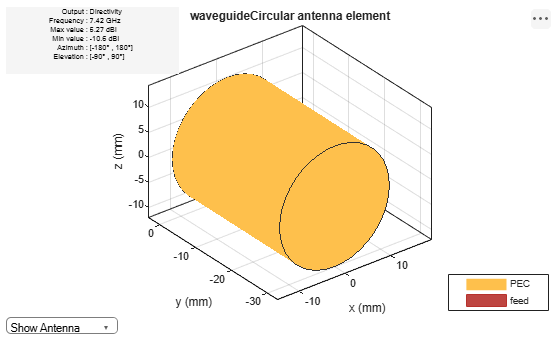Create a circular waveguide with the following dimensions.

```ant = waveguideCircular(Radius=35.7e-3,Height=200e-3,... Feedwidth=26e-3,FeedHeight=34.71e-3,FeedOffset=42.42e-3); show(ant)```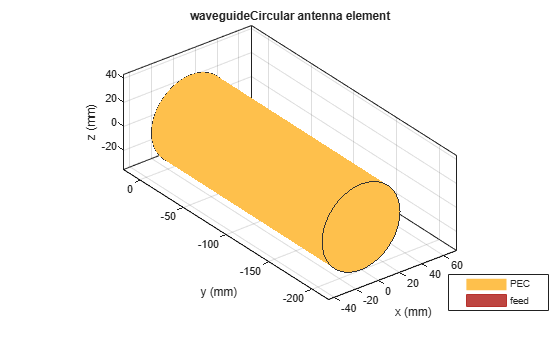Plot the S-parameters and impedance of the waveguide.

```s = sparameters(ant,linspace(2.5e9,4e9,45)); rfplot(s);```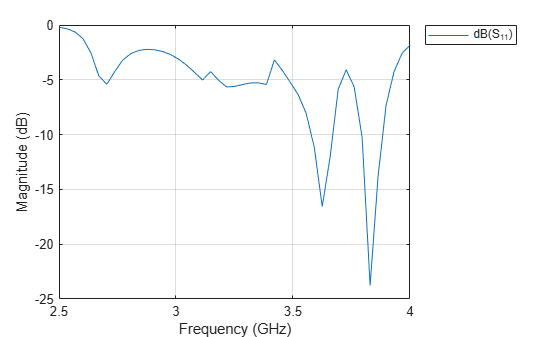```figure impedance(ant,linspace(2.5e9,4e9,45));```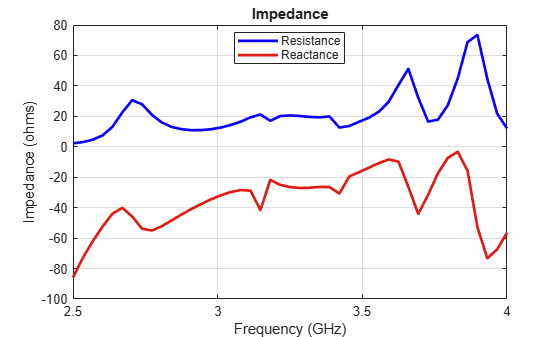Jadhav, Rohini.P, Vinithkurnar Javnrakash Dongre, Arunkumar Heddallikar. "Design of X-Band Conical Horn Antenna Using Coaxial Feed and Improved Design Technique for Bandwidth Enhancement." In International Conference on Computing, Communication, Control, and Automation (ICCUBEA), 1-6. Pune, India: ICCUBEA 2017